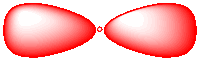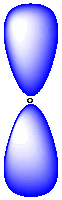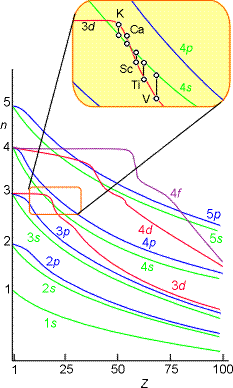# 0.5C: Electronic Configurations - The Aufbau Process

The Aufbau model lets us take an atom and make predictions about its properties. All we need to know is how many protons it has (and how many electrons, which is the same as the number of protons for a neutral atom). We can predict the properties of the atom based on our vague idea of where its electrons are and, more importantly, the energy of those electrons.

How electrons fill in their positions around an atom is called the Aufbau Process (German: "building-up" process). The Aufbau Process is all about keeping electrons at their lowest possible energy and is the direct result of the Pauli Exclusion Principle. A corollary of Coulomb's law is that the energy of an electron is affected by attractive and repulsive forces. The closer an electron to the nucleus, the lower its energy. The closer an electron is to another electron, the higher its energy.

Of course, a basic principle of thermodynamics is that a system will proceed to the lowest energy possible. That means, if an atom has only one electron, the electron will have quantum numbers that place it at the lowest possible energy. It will be as close as possible to the positive nucleus.

If an atom has a second electron, it will also be as close as possible to the nucleus. It could have the same quantum numbers as the first electron, except for spin. There is a trade-off, of course, because those two electrons will be close enough to repel each other. However, if it is a choice between that and taking a position much farther from the nucleus, the second electron will go ahead and pair up. These two electrons are sometimes described as being "in the same orbital;" their first three quantum numbers are the same, so that are probably found somewhere in the same region of space. This first orbital, which has no directional restrictions, is called the 1s orbital.Figure AT4.1. The 1s orbital has no directional preference. Like the yellow tennis ball, it can be found in any direction from the nucleus.

There is only room for one orbital at this distance from the nucleus. A third electron has to occupy another orbital farther away, the 2s orbital. Again, this is a spherical orbital: the electron can be found in any direction. The 2 in 2s means the principal quantum number is two (corresponding to the second general energy level). The s is a code for other quantum numbers; it means the electron can be found in any direction, just like the 1s electrons.

The second energy level is large enough to accommodate additional orbitals, but they are a little farther from the nucleus. These are called the 2p orbitals. They are regions of space along the x, y and z axes. There are three orbitals of this type, and they are just called px, py and pz to remind us that they are orthogonal to each other.Figure AT4.2. The 2px orbital has a directional preference. Like the red tennis ball, it can be found only along one axis.Figure AT4.3. The 2py orbital has a directional preference. Like the red tennis ball, it can be found only along one axis.

Expressed in a different way, an electron with principal quantum number 2 can have four different combinations of its other quantum numbers. These combinations are denoted 2s, 2px, 2py and 2pz. The three 2p combinations are a little higher in energy than the 2s orbital.

A third electron will go into the 2s orbital. It is the lowest in energy. What about a fourth? Does it go into the 2s or a 2p? Once again the pairing energy is not quite as big as the energy jump up to the 2p orbital. The fourth electron pairs up in the 2s orbital.

A fifth electron goes into one of the 2p orbitals. It does not matter which one. We will say it is the px, arbitrarily. A sixth electron again could either pair up in the px, or it could go into the py. But the py level is really the same as the px, just in a different direction. The energy is the same. That means a sixth electron will go into the py rather than pair up in the px, where it would experience extra repulsion.

Note that the p orbitals are often drawn a little differently. For example, p orbitals are usually drawn in a way that shows that they have phase. Either the two lobes are colored differently to show that they are out of phase with each other, or they are shown with one lobe shaded and the other left blank.Figure AT4.5. The 2px orbital shown with phase information.

This pattern is generally followed for all of the elements. The tally of how many electrons are found in each orbital is called the electron configuration. For example, hydrogen has only one electron. Its ground state configuration (that means, assuming the electron has not been excited to another orbital via addition of energy) is 1s1.

On the other hand, an atom with six electrons, such as the element carbon, has the configuration 1s22s22px12py1.

## Periodicity

You may already know that electron configuration is the reason the periodic table works the way it does. Mendelev and others noticed certain elements had very similar properties, and that is because they have very similar electron configurations. Lithium has configuration 1s22s1 and its alkali sister, sodium, has configuration 1s22s22px22py22pz23s1. In both cases, the last electron added is an unpaired s electron. The last electron, or last few electrons, added to an atom generally play a strong role in how the atom behaves. This "frontier" electron is the one at the outer limits of the atom. If the atom is to interact with anything, the frontier electron will encounter the thing first. In contrast, the "core" electrons closer to the nucleus are more protected from the outside.

The order of electrons in an atom, from lowest to highest energy, is:

• 1s
• 2s
• 2p

There are some shortcuts we take with electron configurations. We tend to abbreviate "filled shells" (meaning all the possible orbitals with a given principal quantum number are filled with electrons) and filled "sub-shells" (like 2s or 2p). First of all, in the case of p orbitals, if all the p orbitals are filled, we might just write 2p6 instead of 2px22py22pz2, because there is only one way all the orbitals could be filled. However, we would not necessarily write 2p2 instead of 2px12py1, because we may wish to make clear that the configuration does not involve two electrons in one p orbital at that point, as in 2px22py0.

Also, we dispense with orbital labels entirely to ignore core electrons in a filled shell. For example, instead of writing 1s22s1 for lithium, we can write [He]2s1. Instead of writing 1s22s22px22py22pz23s1 for sodium, we write [Ne]3s1. The [He] means all the electrons found in a helium atom, which is a noble gas. The [Ne] means all the electrons found in a neon atom. A noble gas is an unreactive element with a filled shell: helium, neon, argon, krypton, xenon or radon. The electrons beyond the noble gas shell are called the valence electrons.

principal quantum number 3 actually allows a third set of orbitals. These are called the d orbitals that are a little like p orbitals, but they are two-dimensional rather than one-dimensional. A d electron, for example, might extend along the x axis and the y axis, but not in between the axes.

The d orbitals have five allowed orientations with dxy extending along the x and y axes, dxz extending along the x and y axes (called ), dyz extending along the y and z axes. The other two orbitals extend between the axes instead, dx2-y2 rotated 45 degrees away from one of the other d orbitals and extends between the x and y axes. In the same way, you could imagine an orbital between the x and z and between the y and z axes, but that would make six different orientations. Quantum mechanical rules do not allow that. As a result, two of the possible combinations collapse into a mathematical sum, making just one orbital. We call this one the dz2 orbital.

However, just as the 2p orbitals are higher in energy than the 2s orbitals, the 3d orbitals are higher in energy than the 3p orbitals. The 3d level is very similar in energy to the 4s level. For that reason, calcium's last electrons go into a 4s orbital, not a 3d orbital. Calcium behaves much like magnesium as a result.

The order of electrons in an atom, from lowest to highest energy, is:

• 1s
• 2s
• 2p
• 3s
• 3p
• 4s
• 3d
• 4p

## Reality is Less Simple

The atomic orbital energies do not properly follow this trend as shown pictorially below. For example, the relative energies of the 3d and 4s orbitals switch between calcium and scandium.Energies of Atomic Orbitals as Functions of Nuclear Charge for Neutral Atoms.

### Problem AT4.1.

Write electron configurations for the following elements.

1. oxygen, O
2. sulfur, S
3. silicon, Si
4. nitrogen, N
5. argon, Ar

6. neon, Ne

### Problem AT4.2.

Write abbreviated electron configurations for the following elements.

1. chlorine, Cl
2. calcium, Ca
3. aluminum, Al
4. phosphorus, P

### Problem AT4.3.

Write abbreviated electron configurations for the following elements.

1. iron, Fe
2. copper, Cu
3. mercury, Hg